Click on any of the examples for further details.
Index

Collision concepts

 HyperPhysics***** Mechanics R Nave
Go Back

# Elastic Collision, Equal Masses

For a head-on collision with a stationary object of equal mass, the projectile will come to rest and the target will move off with equal velocity, like a head-on shot with the cue ball on a pool table. This may be generalized to say that for a head-on elastic collision of equal masses, the velocities will always exchange.For a non-head-on elastic collision between equal masses, the angle between the velocities after the collision will always be 90 degrees. The spot on a pool table is placed so that a collision with a ball on the spot which sends it to a corner pocket will send the cue ball to the other corner pocket.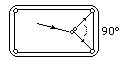Elastic collisions, target at rest Standard examples, elastic collisions Calculation for headon case.
 Role in the moderation of nuclear fission
Index

Collision concepts

Elastic collisions

 HyperPhysics***** Mechanics R Nave
Go Back

# Elastic Collision, Massive Projectile

In a head-on elastic collision where the projectile is much more massive than the target, the velocity of the target particle after the collision will be about twice that of the projectile and the projectile velocity will be essentially unchanged.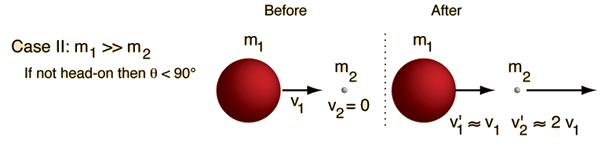For non-head-on collisions, the angle between projectile and target is always less than 90 degrees. This was important in the analysis of the original Rutherford scattering experiment.

This case implies that a train moving at 60 miles/hr which hits a small rock on the track could send that rock forward at 120 miles/hr if the collision were head-on and perfectly elastic. It also implies that if the speed of the head of your golf club is 110 miles/hr, the limiting speed on the golf ball off the tee is 220 miles/hr in the ideal case. That is, the limiting speed of the ball depends strictly on the clubhead speed, and not on how much muscle power you put into the swing.

 Elastic collisions, target at rest Standard examples, elastic collisions Calculation for headon case.
Index

Collision concepts

Elastic collisions

 HyperPhysics***** Mechanics R Nave
Go Back

# Elastic Collision, Massive Target

In a head-on elastic collision between a small projectile and a much more massive target, the projectile will bounce back with essentially the same speed and the massive target will be given a very small velocity. One example is a ball bouncing back from the Earth when we throw it down.In the case of a non-headon elastic collision, the angle of the projectiles path after the collision will be more than 90 degrees away from the targets motion.

 Elastic collisions, target at rest Standard examples, elastic collisions Calculation for headon case.
Index

Collision concepts

Elastic collisions

 HyperPhysics***** Mechanics R Nave
Go Back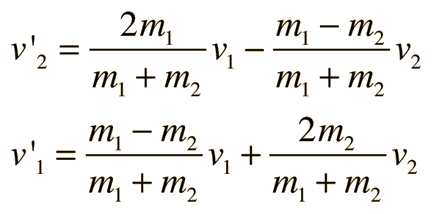can be used to calculate the velocities resulting from any such collision. For the case where the target is at rest (v2 = 0) these relationships reduce to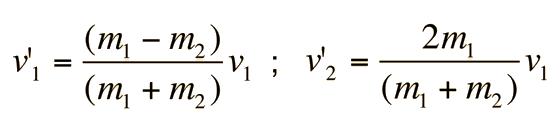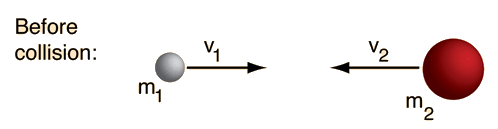Initial conditions:
 m1 =kg, v1=m/s m2=kg, v2=m/s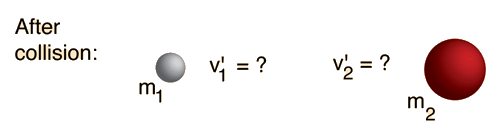Final velocities: v'1= m/s, v'2= m/s
Velocity of approach = m/s
Velocity of separation = m/s

A positive velocity is taken as a velocity to the right in the calculation, so for leftward motion you should enter a negative number. The calculation assumes that a collision took place, but if you get a negative number for the velocity of approach above, this means you chose a scenario where no collision would actually happen.

Index

Collision concepts

Elastic collisions